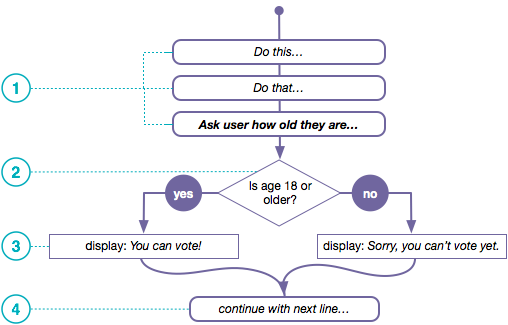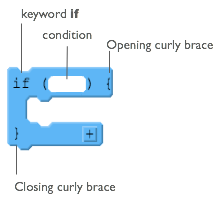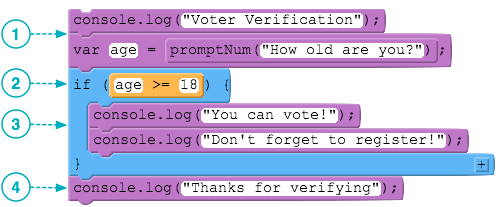# If Statements

## Understanding Program Flow

Programs are said to have a "flow of execution". You start by executing some line of code and then the next and so on.A flow chart is a common visual that's used to represent the various paths of execution that your program might take. Many people use them to help plan.

1. This flow chart depicts a program executing one line after another until it gets to a point where it needs to make a decision.

2. In order to determine which path to take you state some condition. It should be a Boolean expression - something that evaluates to true or false. Here we have a simple comparsion of two values: the person's age and the number 18.

3. The program does one thing if the condition is true, and something else if the condition is false.

4. The pr

## How If-statements work`if` statements are the lines of code you need to change the flow while you're program is running. You can write code that makes a decision that determines which lines of code should be run next.

At the right is a diagram showing the elements of a basic `if` statement in JavaScript.

There are two basic parts to an if-statement.

1. A condition to be evaluated (A Boolean expression that evaluates to true or false)
2. Code that should run if the expression was true - enclosed in curly braces

## A worked example1. Program executes line by line as you would expect. It displays a message, then prompts the user to enter a number. Whatever the user types will be stored in the variable `age` and then proceeds to the next line...

2. When the `if` statement is encountered the first thing it does is evaluate the condition in the parentheses. It checks to see if, at this point in the program, the value in a variable `age` is greater than or equal to 18. If it is then we say the expression "returns true". Otherwise it returns false.

3. These console.log statements will only execute if the expression was true. The curly braces surround all of the code that should be executed if, and only if, the expression was true. Otherwise, the entire section of code encapsulated in the if statement is skipped.

4. Execution picks up here, on the first line after the closing curly brace of the if-statement. This line will always execute, but notice that if the user entered an age less than 18, the entire if statement would be skipped, and it would just say "Thanks for verifying". (We'll fix this awkwardness soon).

Program can continue a single thread of execution after the condition as well.

Found a bug in the documentation? Let us know at documentation@code.org